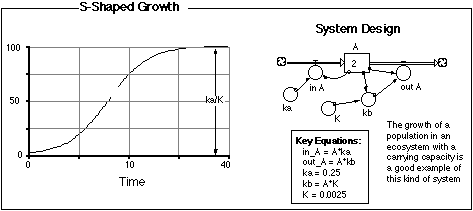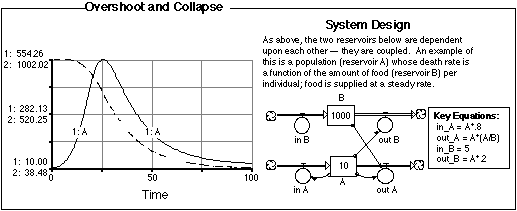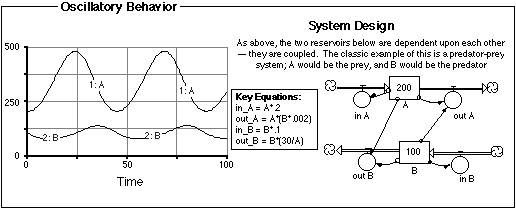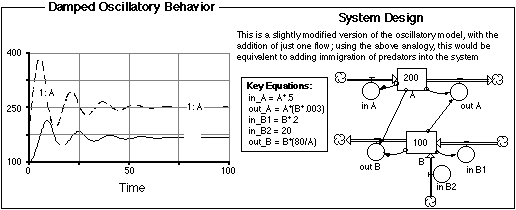## Common System Designs and Behaviors

There are probably an infinite number of models of dynamics systems, but some common design elements or system structures are found in a great number of models. When we venture off into world of modeling, it will be helpful to be familiar with some of these common designs and it will be especially helpful develop a sense of what types of behaviors are associated with these designs.

The series of figures below illustrate these comon system designs in the form of very simple STELLA models, accompanied by graphs that show the behavior or evolution of these systems over time, and the basic equations used in the models. In each case, I mention a real-life system that similar to these models.Figure 2.14 Linear Growth and Decay. The key to this system design is that the flows are defined as constants; this results in a very simple, easy-to-predict behavior characterized by constant rates of change.Figure 2.15 Exponential Growth and Decay. These are extremely common design elements that are sometimes referred to as first-order kinetic equations, but in simpler terms, they represent growth or decay (draining) processes where the rate of change is a fixed percentage of the reservoir involved in the flow. Exponential growth represents a classic form of positive feedback, yielding a runaway behavior, while exponential decay represents a negative feedback mechanisms that has a stabilizing effect.Figure 2.16 S-Shaped Growth. This system design is especially common in models of population growth that is limited by some resource. In this type of a system, one of the flows is defined as a percentage of the reservoir, but that percentage changes as the amount in the reservoir changes. This kind of a system design has a sort of built-in limit, determined by the rate constants, as shown in the figure. Interestingly, this sytem structure can lead to chaotic behavior as we will see in a later chapter on population growth.Figure 2.17 Overshoot and Collapse. This system design represents a variation on the system shown in Figure 2.16. In this case, the limit to growth, represented by reservoir B is declining as reservoir A increases. Reservoir A grows exponentially and shoots past its limit and as a result, the limit decreases more and more, fueling a collapse of A until it reaches a steady-state at a very low level.Figure 2.18 Oscillatory Behavior. This type of system design represents what is called a coupled system since the change of each reservoir is dependent on how its companion reservoir is changing. These systems often lead to an oscillation, creating cycles that do not have an external control. The oscillation arising from these coupled reservoirs is very different from the kind of oscillation forced onto a system by some external control - something that is a sinusoidal function of time.Figure 2.19 Damped Oscillatory Behavior. This is a variation of the system shown in Figure 2.18 and is common in any environment where friction or some other form of energy loss is a factor. Interestingly, it is also a consequence of migrations in systems of coupled populations.